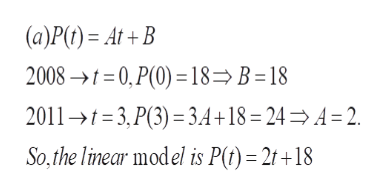# Linear models can be used to predict buying habits of consumers. Suppose that a survey found that in 2008,18% of the consumers surveyed in a group bought designer frames for their eyeglasses. In 2011, the percentageclimbed to 24 %a. Assume the percentage of people buying frames is a linear function of time, and find an equation for thepercentage of people buying designer frames. Let t correspond to the number of years since 2008P(t)b. Use your equation to predict the percentage of people buying designer frames in 2014The model predicts the percentage of people buying designer frames in 2014 will be |c. Use your equation to predict when the percentage of people who buy designer frames will reach 50 %The model predicts in the year1, 50% of people will buy designer framesd. Do you think you can use this model to predict the percentage of people buying designer frames in the year2051? Why or why not?

Question
21 views
check_circle

Step 1

To set up linear equation(s) corresponding to the given data

Step 2

In this problem, the independent variable is t= the number of years after 2008, and the dependent variable is the percentage P(t). A linear model connecting these two variables will have an equation of the form P(t) = At + B, for some constants to be determined

Step 3help_outlineImage Transcriptionclose(a)P(t) At+B 2008t=0, P(0) 18= B = 18 2011-3, P(3) 3.4+18 =24 = A = 2 So,the line ar model is P(f)= 2t +18 fullscreen

### Want to see the full answer?

See Solution

#### Want to see this answer and more?

Solutions are written by subject experts who are available 24/7. Questions are typically answered within 1 hour.*

See Solution
*Response times may vary by subject and question.
Tagged in

### Algebra